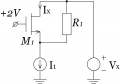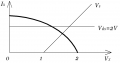# Problem with a problem from Razavi's book

#### IgorBurmistrov

Joined Feb 4, 2017
5
Hello everyone!

I currently trying to solve problem 2.6.C from Razavi's "Design of analog CMOS IC". (Circuit attached to post) The goal is to plot current Ix (drain current), while Vx changes from 0 to 3V.

Due to the current source, problem gets too hard for me and I look in to the solutions. Author of its gets that while Vx changes, voltage at the positive port of CS equal to 0, but I think thats is wrong, because if so when Vx = 0, current don't flow at all. So CS need to make voltage at the positive port so negative that voltage drop across the resistor R1 and the MOS M1 gives the I1 current. When Vx grows up, voltage at the positive port also changes in any direction, but not stays in initial state or 0.

So. Maybe I wrong with this and dont understande how CS works?Last edited by a moderator:

#### Jony130

Joined Feb 17, 2009
5,244
For Vx = 0V the current will flow. Without the MOS for Vx = 0V the voltage at the MOS source is -I1*R1. So, for sure for Vx = 0V the MOS transistor will be ON, as long as Vth < 2V+I1*R1 and it will work in triode mode because Vds < (Vg - Vth)

#### IgorBurmistrov

Joined Feb 4, 2017
5
Yes I think in the similar manner (negative Vs). So, it's not was wrong? Good, bit it's make problem more difficult.

Case when Vx = 0. Voltage drop across the R1 is Vds for MOS and its equal to -I1*R1. Minus sign is because current need to flow into CS, while Vx = 0 (or less than 0, for common case). And, if so, MOS is open, if Vgs = 2V - (-I1*R1) = 2V + I1*R1 greater than Vth. Now, if MOS is open than, not all the current will flow through R1, but only Ir = I1-Ix and Vds now is voltage drop of I1 across R1 and M1.

Also M1 may work in saturation region, when Vds > Vgs - Vth, but Vds = Vs and Vgs = 2 - Vs, so M1 in sat., when Vs < 2 - Vs - Vth or 2Vs < 2 - Vth. So it's not work in triode for sure.

#### Jony130

Joined Feb 17, 2009
5,244
At first try analysis this two cases:
1). Vx = 0V I1 = 10mA; R1 = 100Ω and for the MOS Vth = 1V , λ = 0.1 and K = 1

2) To turn off the MOS we need Vs >1, Vx > (2V - Vth = 1V across the current source) + ( I1*R1) = 1V +1V = 2V

#### IgorBurmistrov

Joined Feb 4, 2017
5
Ok.

Voltage drop across $$V_R_1 = I_1 \cdot R_1 = 100 mA \cdot 100 \Omega = 1 V$$
So we have $$V_s = -1 V$$, $$V_d = 0 V$$ and $$V_g = 2 V$$ therefore $$V_{ds} = 1 V$$ and $$V_{gs} = 3 V$$.
$$V_{sat} = V_{gs} - V_{th} = 2 V > V_{ds}$$, so M1 is in triode region.
When $$V_x {\to} V_{dd} = 3$$ voltage drop at $$R_1$$ as $$V_{ds}$$ will remain, but $$V_{gs}$$ will decreace, therefore we have plot of $$I_x$$ as at attached pic.Moderatots note: shown picture full size

Last edited by a moderator:

#### IgorBurmistrov

Joined Feb 4, 2017
5
My mistake - Ix will have linear decreace not quadratic.

#### MrAl

Joined Jun 17, 2014
8,500
Hello everyone!

I currently trying to solve problem 2.6.C from Razavi's "Design of analog CMOS IC". (Circuit attached to post) The goal is to plot current Ix (drain current), while Vx changes from 0 to 3V.

Due to the current source, problem gets too hard for me and I look in to the solutions. Author of its gets that while Vx changes, voltage at the positive port of CS equal to 0, but I think thats is wrong, because if so when Vx = 0, current don't flow at all. So CS need to make voltage at the positive port so negative that voltage drop across the resistor R1 and the MOS M1 gives the I1 current. When Vx grows up, voltage at the positive port also changes in any direction, but not stays in initial state or 0.

So. Maybe I wrong with this and dont understande how CS works?

View attachment 119886

Hi,

Not sure if i understand you right, but looking at the schematic it sounds like you are saying that the top of the current source must go negative at some point, like when Vx=0. The context however is a "CMOS IC" which typically has no negative power supply. If this is a typical CMOS gate then the voltage at the top of the current source would never be able to go negative simply because there is no internal mechanism that would allow the device to create a negative voltage.

#### Russmax

Joined Sep 3, 2015
82
MrAl, this is not a circuit in an IC, but a circuit in a textbook. So the voltage at the top of the current source can be any value that makes the math work. What's more, I've designed ICs where voltages in the circuit were as low as -130 V. The substrate doesn't have to be ground, and even if it is a grounded substrate, isolated P-wells can be formed in which source voltages are << 0.

So, when Vx = 0, the transistor is most fully on (Vgs is largest). As Vx rises, so does Vs, reducing Vgs and M1 current, thereby increasing R1 current. Without knowing I1, Vth, uCox, etc. I don't know what the thresholds are, but that's the trend.

#### MrAl

Joined Jun 17, 2014
8,500
Hi again,

Ok then this sounds like one of those problems where you must contact the author to get the understanding of what assumptions they are allowing.

For example, with just R1 in the circuit (MOSFET off) it is very obvious that the constant current source will create a negative voltage at the top of it (schematically the top). If however the mosfet is assumed to be fully on (zero resistance) with +2v gate to source voltage, then the mostfet could be said to be shorting Vx to the top of the current source, and since Vx is zero then so is the top of the current source. I say this because if we allow even 1mOhm for the mosfet Rds we will see at least a small negative voltage at the top of the current source. So i think you have to go back to the author of this circuit and ask the for an explanation.